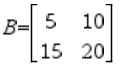# Knowledge Base

## Solution 33871: Finding the Reduced Row Echelon Form of a Matrix on a TI-36X Pro.

### How do I find the reduced row echelon form of a matrix on a TI-36X Pro?

To find the reduced row echelon form of a matrix, input the matrix, exit the matrix editor, and then paste the rref() function on the home screen with the name of the matrix.

The example below will demonstrate this procedure:

Example: Find the rref( of matrix B.To enter the matrix:

1) Press [2nd] [matrix].
2) Scroll over to Edit and scroll down to B.
3) Press [enter].
4) Select 2 for ROWS and 2 for COLUMNS and scroll to OK and press [enter].
5) Input the matrix entries, pressing enter after each entry. For example,  [enter]   [enter]   [enter]   [enter].
6) Exit the matrix editor by selecting [2nd] [quit].

To find the reduced row echelon form using the rref( function:

1) Press [2nd] [matrix].
2) Scroll to MATH.
3) Press 5: rref reduced.
4) Press [2nd] [matrix].
5) Press 2: [B] 2x2.
6) Press [)] [enter] to display the answer.